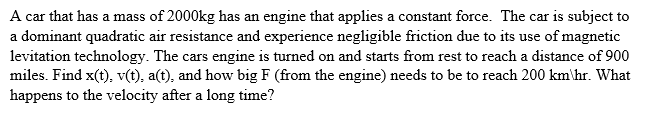# Finding Force Needed for 200 km/hr

• Ashley1nOnly

## Homework StatementLooking for F for 200 km/hr

F=ma

## The Attempt at a Solution

F-cv^2 = ma
F= ma +cv^2

v=200 km/hr

F= ma +c(200 km/hr)^2

so now I need to find a, from previous work I found that

t= (m/c)*(1/2 vter)*(ln[ (vter +v)/(vter-v)])

next I would solve for v and take the derivate to get a.

but I know that

v^2 =v0^2+2a( x -x0)

where

a= v^2-v0^2 / 2(x-x0)

I know that v=2000 km/ hr
v0=0 since it was at rest
x= final distance
x0=0 since it starts at the origin

#### Attachments

Last edited:
Thanks Charles and SammyS.

@Ashley1nOnly What makes you think you will understand what 69 posts before didn't achieve, which btw. is still open?